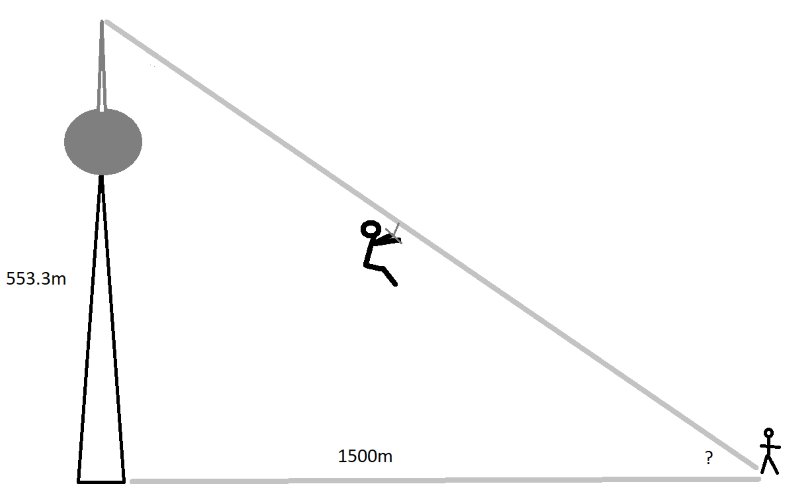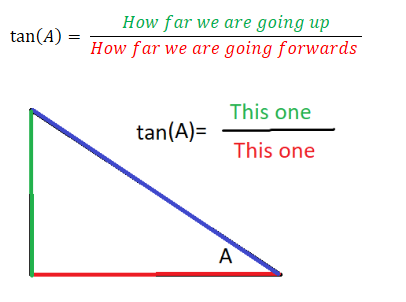top of page
Search

# Sine and Cosine TutorialKey concepts:

Trigonometry

Sine and cosine

Geometry

Look, we get it; trigonometry kind of sucks. As if regular geometry wasn't bad enough by itself, you are now being asked to remember all of these cosine and sine things (whatever they are). Let's get the basics straight:

Question 1:

"You point a laser range finder towards the tip of the CN tower at an angle of 70 degrees. It tells you that the tip is 588.8m away from where you are standing. Determine the height of the CN tower and how far away it is."

To solve this question, we need the following:

1. A sketch of the situation we are trying to analyze

2. Some equations that relate different quantities in the problem to each other.

3. A way to double check if the answer makes sense.

1. A sketch of the situation we are trying to analyze

The sketch below shows what we know (the direct distance from you to the tower tip) and the angle you are holding the laser range finder at. It also shows what we want to find (the height of the tower and the distance to it).So what on earth do we do now? To solve 2. we need some trigonometry:

2. Some equations that relate different quantities in the problem to each other.

Let us first think about how cosine and sine are even defined:(Yes, I know this is a dumb way to remember it, but trust me; it actually works!)

In the problem we are trying to solve, we can plug in the numbers and isolate d:So we should be around 200m away from the tower.

What about the height of the tower?(Yes, I know this is an even dumber way to remember it, but sometimes dumb solutions are the smartest ones.)

Let us try to plug in the numbers and solve for the height:Now for 3.

3. A way to double check if the answer makes sense.

The no-brainer solution is just to look up the height of the CN-tower. According to Wikipedia it is 553.3m tall. That is a good sign our answer is correct.TIP: I know it seems silly to look up a wikipedia page like this, but double checking answers and thinking critically about your results is extremely important. I cannot tell you how many times I have gone through a lengthy calculation only to get the wrong result because I was too lazy to verify my answer - goodbye extra marks!

Question 2:

"You are standing 100m away from the base of the CN-Tower. You look up at a 79.75 degree angle and stare directly at its tip. Use this information to determine the height of the CN-Tower."

To solve this question, we need the following:

1. A sketch of the situation we are trying to analyze

2. Some equations that relate different quantities in the problem to each other.

3. A way to double check if the answer makes sense.

Let us get started with 1.:

1. A sketch of the situation we are trying to analyzeThe first thing we notice is that the tip of the tower, its base and the place we are standing creates a triangle. What kind of triangle is this? It must be a right angle triangle!Making a drawing always makes the problem a lot easier! Anyways, let us get started with 2.

2. Some equations that relate different quantities in the problem to each other.

Right now, we know 2 out of 3 angles in the triangle. One is 79.75 degrees, the other is 90 degrees, so the final one must be 180-90-79.75=10.25 degrees. We know all of the angles, but only one of the sides of the triangle. Luckily, we can use the Law of Sines:In the present case, we could write down the following expression and solve for h:Very nice!

DOUBLE CHECK: If we had typed the numbers into our calculator and gotten the result 127m would you believe it without a doubt? On one hand, 127m is tall for a building, but not that tall for something considered a major landmark in a huge city with lots of skyscrapers. We suspect that we made a mistake in the calculation, but what exactly went wrong?

A common mistake when taking the sine of an angle is to write the angle in degrees, when the calculator expects it to be in "radians".

Wrong calculation:If you can't figure out how to tell your calculator to switch between radians and degrees, here is a useful trick to convert between them manually:To check if the calculator takes radians or degrees, simply punch in:

## SIN(90)

If the result is 1, then it reads 90 as being in degrees.

If the result is 0.027, it thinks 90 is in radians.

Question 3:

"You want to run a zip-line from the top of the CN tower to a point located 1.5 km away from its base. Determine the angle of this zip-line relative to the ground."

Take a wild guess what we need to solve this problem? That's right:

1. A sketch of the situation we are trying to analyze

2. Some equations that relate different quantities in the problem to each other.

3. A way to double check if the answer makes sense.

Let get right to solving 1.

1. A sketch of the situation we are trying to analyzeBefore we go any further, let us be clear: We are interested in the unknown angle labelled "?". Does this angle become bigger or smaller if we double the height of the tower?

Clearly, if we stack another CN tower on top of the first one and run a zip-line to the same point, the angle is going to be closer to 90 degrees. We can also get a bigger angle by moving the end-point closer to the base of the tower.

Similarly, if we cut the tower in half, the angle will be closer to 0 degrees. Moving the point further away gives us the same result.

But enough thought experiments. Let's take care of 2.

2. Some equations that relate different quantities in the problem to each other.

To solve this problem, we need the "third" trigonometric function:Basically, you can think of "tan" as telling you how steep the slope of a line is. If tan is big, the slope is really steep.

Let us try to plug in the numbers:One way to interpret this result is:

"Every time we go 100m forwards, the height of the zip-line above the ground increases by 37m".

Notice that 0.37 is not the angle in either degrees or radians! It just tells us something about the steepness.

Ok, that is all well and good, but how do we get the actual angle? Well, we just use the inverse function of tan:Does that make sense? We still need:

3. A way to double check if the answer makes sense.

Let us think about it this way: If we chose to end the zip-line exactly 553.3m away from the base of the tower, then it would clearly be at a 45 degree angle.

Remember what we said earlier about making the tower shorter or the distance to it greater?

"Similarly, if we cut the tower in half, the angle will be closer to 0 degrees. Moving the point further away gives us the same result."

If the distance is 553.3m, then the angle is 45 degrees. By the logic above, if we increase the distance to 1500m, then the angle should decrease. Indeed if we choose 1500m we get a smaller angle of 20.3 degrees.

Conclusion:

If you forget everything else, try to remember these definitions of cosine, sine and tangent: